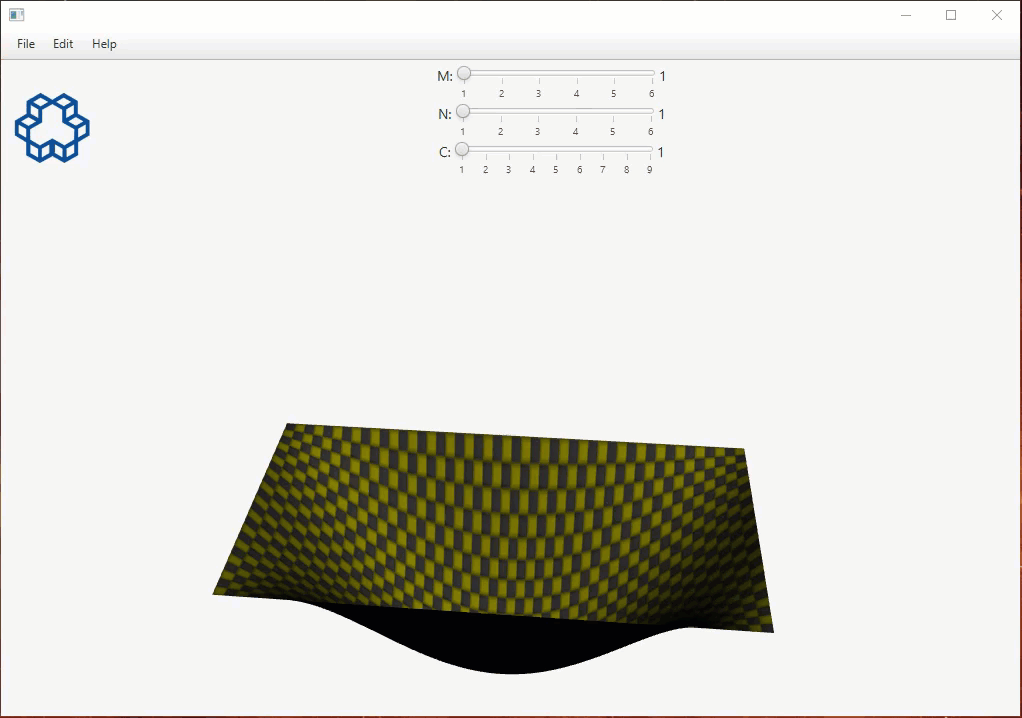Rectangular Membrane

## Vibration of Rectangular MembraneSolutions of this equation describe propagation of disturbances out from the region at a fixed speed in one or in all spatial directions, as do physical waves from plane or localized sources; the constant c is identified with the propagation speed of the wave. This equation is linear. Therefore, the sum of any two solutions is again a solution: in physics this property is called the superposition principle.
The wave equation alone does not specify a physical solution; a unique solution is usually obtained by setting a problem with further conditions, such as initial conditions, which prescribe the amplitude and phase of the wave. Another important class of problems occurs in enclosed spaces specified by boundary conditions, for which the solutions represent standing waves, or harmonics, analogous to the harmonics of musical instruments.
The wave equation, and modifications of it, are also found in elasticity, quantum mechanics, plasma physics and general relativity.